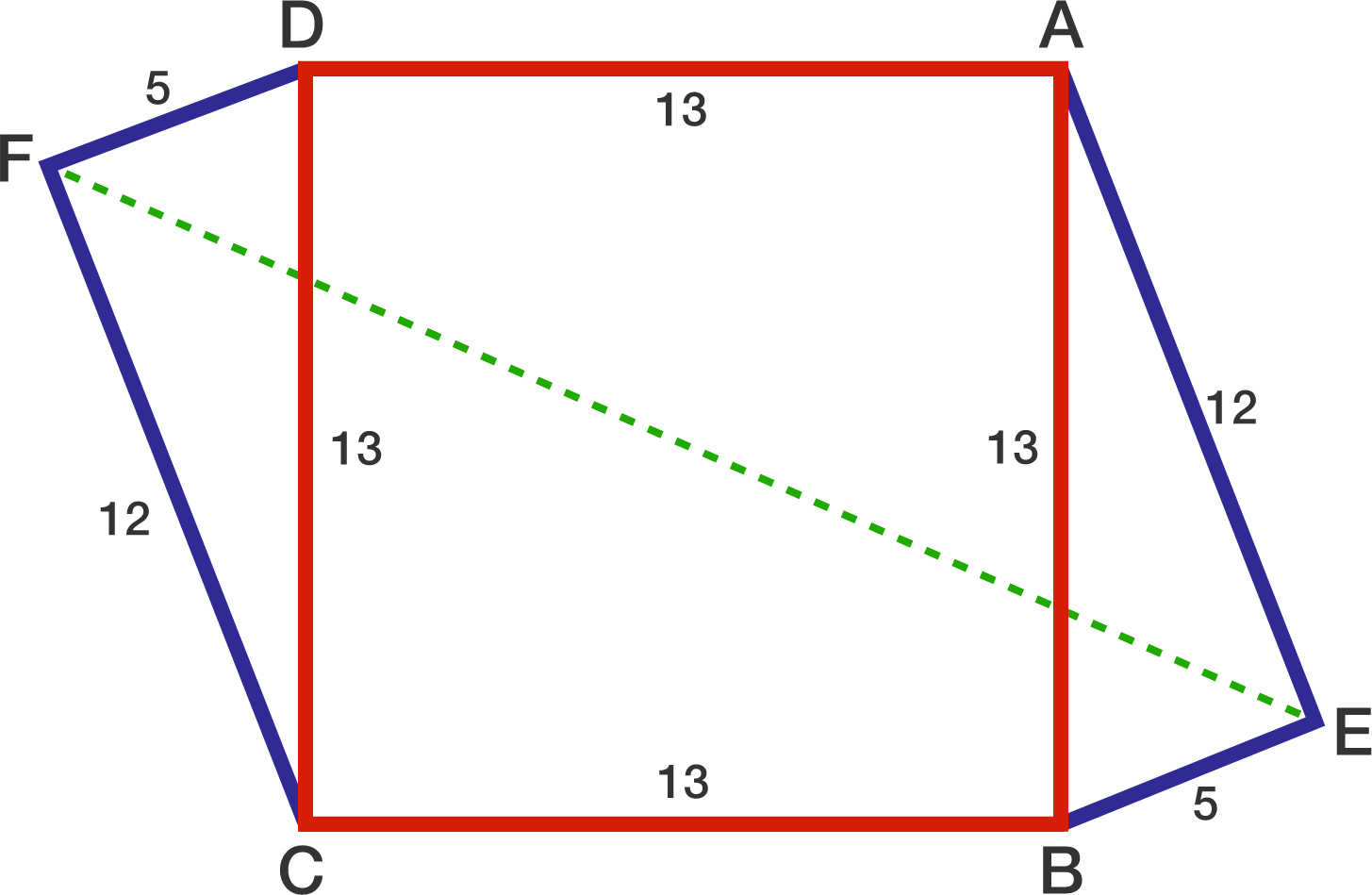# 5-12-13 and square!

Geometry Level 2$ABCD$ is a square with $AB=13$. Points $E$ and $F$ are exterior to $ABCD$ such that $BE=DF=5$ and $AE=CF=12$.

If the length of $EF$ can be represented as $a\sqrt b,$ where $a$ and $b$ are positive integers and $b$ is not divisible by the square of any prime, then find $ab$.

×